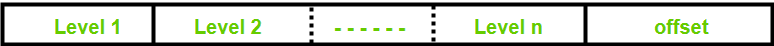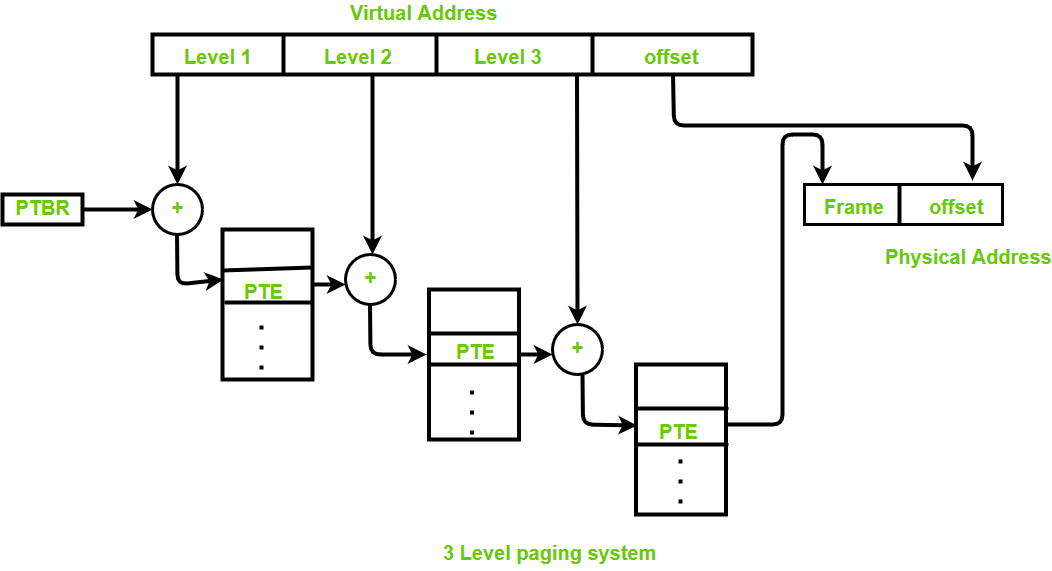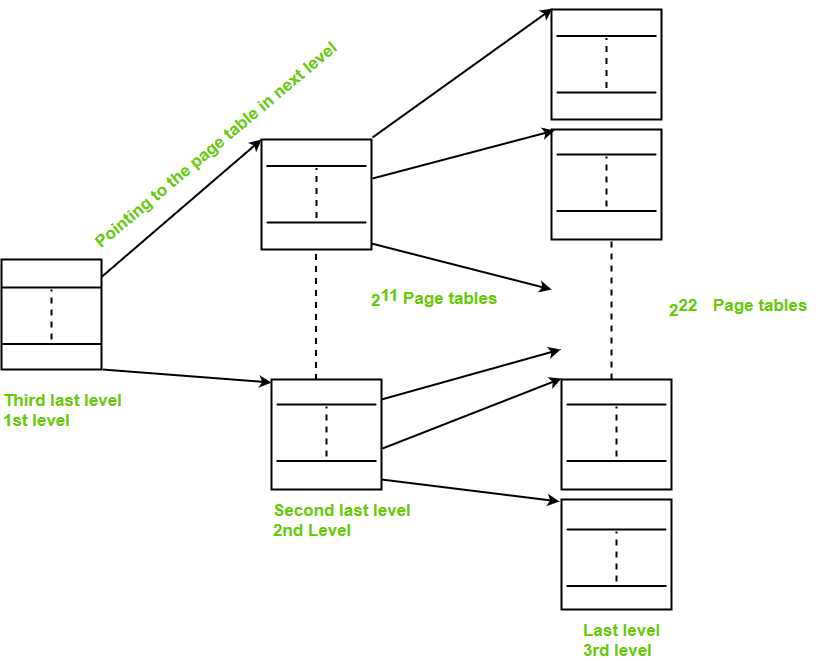# Multilevel Paging in Operating System

• Difficulty Level : Easy
• Last Updated : 13 Jun, 2022

Prerequisite – Paging

Multilevel Paging is a paging scheme that consists of two or more levels of page tables in a hierarchical manner. It is also known as hierarchical paging. The entries of the level 1 page table are pointers to a level 2 page table and entries of the level 2 page tables are pointers to a level 3 page table and so on. The entries of the last level page table store actual frame information. Level 1 contains a single-page table and the address of that table is stored in PTBR (Page Table Base Register).

Why it is required?

If the frame size of the main memory is smaller than the page size and the process cannot fit that way then we divide the pages into further pages, and this concept is known as multilevel paging.In multilevel paging whatever may be levels of paging, all the page tables will be stored in the main memory. So it requires more than one memory access to get the physical address of the page frame. One access for each level is needed. Each page table entry except the last level page table entry contains the base address of the next level page table.Reference to actual page frame:

• Reference to PTE in level 1 page table = PTBR value + Level 1 offset present in virtual address.
• Reference to PTE in level 2 page table = Base address (present in Level 1 PTE) + Level 2 offset (present in VA).
• Reference to PTE in level 3 page table= Base address (present in Level 2 PTE) + Level 3 offset (present in VA).
• Actual page frame address = PTE (present in level 3).

Generally, the page table size will be equal to the size of the page.

Assumptions:
Byte addressable memory and n is the number of bits used to represent virtual address.

Important formula:

```Number of entries in page table:
= (virtual address space size) / (page size)
= Number of pages

Virtual address space size:
= 2n B

Size of page table:
<>= (number of entries in page table)*(size of PTE) ```

If page table size > desired size then create 1 more level.

Extra memory references to access address translation tables can slow programs down by a factor of two or more. Use translation look aside buffer (TLB) to speed up address translation by storing page table entries.

Example:
Q.Consider a virtual memory system with physical memory of 8GB, a page size of 8KB, and 46-bit virtual address. Assume every page table exactly fits into a single page. If page table entry size is 4B then how many levels of page tables would be required.

Explanation:

```Page size = 8KB = 213 B
Virtual address space size = 246 B
PTE = 4B = 22 B

Number of pages or number of entries in page table,
= (virtual address space size) / (page size)
= 246B/213 B
= 233 ```

Size of page table,

```= (number of entries in page table)*(size of PTE)
= 233*22 B
= 235 B ```

To create one more level,

```Size of page table > page size

Number of page tables in last level,
= 235 B / 213 B
= 222 ```

The base address of these tables is stored in page table [second last level].```Size of page table [second last level]
= 222*22B
= 224B ```

To create one more level,
Size of page table [second last level] > page size

```Number of page tables in second last level
= 224B/213 B
= 211 ```

The base address of these tables are stored in page table [third last level]

```Size of page table [third last level]
= 211*22 B
= 213 B
= page size ```

∴ 3 levels are required.

My Personal Notes arrow_drop_up